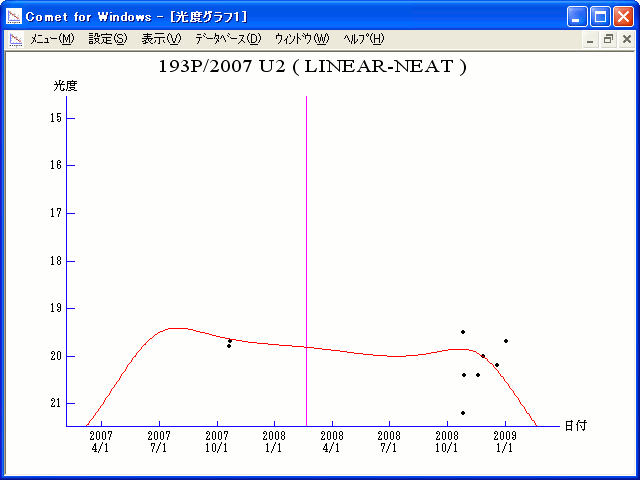# \$B%j%K%"(B-\$B%K!<%HWB@1(B

193P/LINEAR-NEAT (2008)###\$B%W%m%U%#!<%k(B

 \$BId9f(B 193P/2007 U2 \$B8!=PF|(B 2007\$BG/(B10\$B7n(B21\$BF|(B \$B8!=P8wEY(B 19.8\$BEy(B \$B8!=P K. Sarneczky and L. L. Kiss (Siding Spring)

###\$B50F;MWAG(B

```                    Epoch = 2008 Feb. 24.0 TT
T = 2008 Feb. 20.4955 TT         Peri. =   8.2860
e = 0.395943                     Node  = 335.2570  2000.0
q = 2.155746 AU                  Incl. =  10.7041
a =  3.568779 AU    n = 0.1461923    P =   6.742 years
```

###\$B8wEYJQ2=(B

```        m1 = 14.0 + 5 log\$B&\$(B + 10.0 log r
```##### \$B50F;MWAG\$O!"(BIAUC 8885\$B\$K7G:\\$5\$l\$?\$b\$N\$G\$9!#(B \$B8wEY%0%i%U\$O(BComet for Windows\$B\$G:n@.\$7\$?\$b\$N\$G\$9!#(B